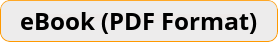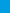# TASC Math Prep 2020 A Comprehensive Review and Step-By-Step Guide to Preparing for the TASC Math TestLooking for a perfect prep book to help you review all TASC Math concepts and topics right from scratch? If so, then you are in the right place. TASC Math Prep 2020 is the only book you will ever need to learn TASC Mathematics topics and prepare for the TASC Math Test.\$20.99 \$15.99
-24% only \$20.99 \$15.99
Rated 5.00 out of 5 based on 1 customer rating

Bulk Orders: Need a license for this book?

### Also included in:

TASC Math Prep 2020 covers basic mathematics concepts including integers, percent, fractions, mixed numbers, ratios, proportions, etc. to more advanced topics such as algebra equations and inequalities, monomials and polynomials, linear and quadratic, three-dimensional figures, statistics, and probability, etc.

Whether you are intimidated by mathematics and you left school years ago, or even if you were receiving A in your high school mathematics classes, this book can assist you incorporate the best and the most effective method and the right strategies to succeed on the TASC Math test. TASC Math Prep 2020 offers a winning formula and the most powerful methods for learning basic Math topics and it is a breakthrough in Mathematics learning.

Each chapter of the book provides many examples and detailed solutions and explanations to help you learn TASC math topics in an effective way. TASC Math Prep 2020 was created by Test Prep experts and it goes into detail to cover all of the content likely to appear on the TASC Math test. There are hundreds of exercises and activities to help you understand each TASC mathematics topic thoroughly. Two full length and realistic TASC Math practice tests at the back of the book measure your readiness for the test and you will find out what score you will be able to receive on a real TASC Math test.

Get a copy of this book and simply follow the instructions in the book to beat the TASC Math test.

1.